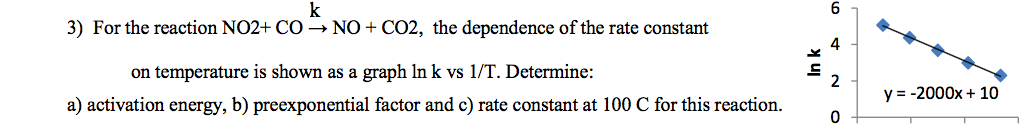# k3) For the reaction NO2+ C0 → NO + CO2, the dependence of the rate constant4on temperature is shown as a graph In k vs 1/T. Determine:2y = -2000x + 10a) activation energy, b) preexponential factor and c) rate constant at 100 C for this reaction.In k

Question
1 viewshelp_outlineImage Transcriptionclosek 3) For the reaction NO2+ C0 → NO + CO2, the dependence of the rate constant 4 on temperature is shown as a graph In k vs 1/T. Determine: 2 y = -2000x + 10 a) activation energy, b) preexponential factor and c) rate constant at 100 C for this reaction. In k fullscreen
check_circle

Step 1

The graph lnk vs 1/T is shown below.

Step 2

The equation of line is shown below.

Step 3

In the graph y-axis corresponds to ln k and x-axis corresponds to 1/T. Therefore, the ...

### Want to see the full answer?

See Solution

#### Want to see this answer and more?

Solutions are written by subject experts who are available 24/7. Questions are typically answered within 1 hour.*

See Solution
*Response times may vary by subject and question.
Tagged in
ScienceChemistry

### Physical Chemistry